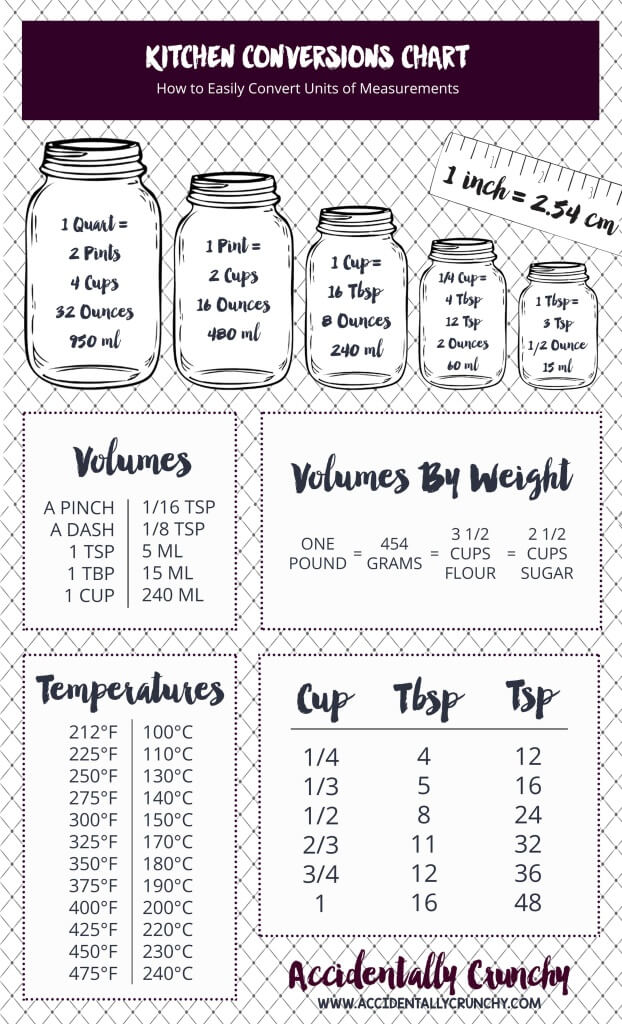# Cooking Measurement Conversions Guide

If you enjoy cooking, you know that following a recipe often requires converting measurements. Whether you are working with international recipes or need to adjust the measurements to fit your needs, having a reliable measurement conversions guide is essential. This guide will provide you with the necessary information to convert common cooking measurements, ensuring your dishes turn out just right every time.

When it comes to converting measurements in the kitchen, accuracy is crucial. One of the most common conversions is converting between ounces (oz), pounds (lb), and grams (g). Here is a helpful conversion table:

Measurement Ounces (oz) Pounds (lb) Grams (g)
1 ounce (oz) 1 0.0625 28.35
1 pound (lb) 16 1 453.59
1 gram (g) 0.0353 0.0022 1

In addition to weight conversions, you may also need to convert volume measurements. Here are some common conversions for liquid ingredients:

• 1 teaspoon (tsp) = 5 milliliters (ml)
• 1 tablespoon (tbsp) = 15 milliliters (ml)
• 1 fluid ounce (fl oz) = 30 milliliters (ml)
• 1 cup = 240 milliliters (ml)

Having a comprehensive measurement conversions guide at your fingertips will save you time and effort in the kitchen. Whether you need to convert weight or volume measurements, this guide will help you achieve consistent and accurate results in your cooking.

## Understanding Cooking Measurement Units### 1. Volume MeasurementsVolume is one of the most commonly used measurement units in cooking. It refers to the amount of space occupied by a substance and is typically measured in milliliters (ml) or liters (L), especially in recipes where liquids are involved. Cups and teaspoons are also commonly used in cooking measurements:

• 1 cup = 240 ml
• 1 tablespoon = 15 ml
• 1 teaspoon = 5 ml

### 2. Weight Measurements

Weight measurements are crucial when it comes to measuring ingredients accurately. Unlike volume measurements, weight is not affected by the type of substance being measured. The most commonly used weight measurement unit is the gram (g), although some recipes also use ounces (oz) or pounds (lb). Here are some commonly used weight conversion factors:

• 1 gram = 0.035 ounces
• 1 kilogram = 2.205 pounds

### 3. Temperature Measurements

Temperature measurements are important for cooking and baking, as they determine how ingredients will react. The two most commonly used temperature scales are Celsius (°C) and Fahrenheit (°F). Conversion formulas exist for converting between the two scales:

Celsius (°C) Fahrenheit (°F)
0 32
100 212
Converting Celsius to Fahrenheit:
(°C × 9/5) + 32 °F
Converting Fahrenheit to Celsius:
(°F – 32) × 5/9 °C

## Converting between Metric and Imperial Units

Here are some common conversions between metric and imperial units:

• 1 gram (g) = 0.035 ounces (oz): This conversion is useful when measuring small quantities of ingredients like spices and herbs.
• 1 kilogram (kg) = 2.205 pounds (lb): This conversion is commonly used for larger quantities, such as meat or flour.
• 1 liter (l) = 0.264 gallons (gal): This conversion is helpful when dealing with liquids, like water or milk.
• 1 milliliter (ml) = 0.034 fluid ounces (fl oz): This conversion is handy for measuring small amounts of liquid ingredients, such as vanilla extract or lemon juice.

It’s important to note that when converting between metric and imperial units, it’s advisable to use a kitchen scale or measuring cups and spoons specifically designed for the system you are working with. This ensures accuracy and eliminates any confusion that might arise from using different measurement tools.

## Converting between Volume and Weight Measurements

Converting between volume and weight measurements depends on the density of the ingredient. Density is the amount of mass per unit volume. Ingredients with different densities will have different weight-to-volume ratios, meaning that the same volume of two ingredients may have different weights.

### Converting from Volume to Weight:To convert from volume to weight, you need to know the density of the ingredient. If the density is not provided in the recipe or packaging, you can refer to conversion charts that provide average densities for common ingredients. Once you know the density, you can use the following formula:

Weight (in grams) = Volume (in milliliters) x Density (in grams per milliliter)

For example, if a recipe calls for 250 milliliters of milk and the average density of milk is 1.03 grams per milliliter, the weight of the milk would be:

Weight = 250 ml x 1.03 g/ml = 257.5 grams

### Converting from Weight to Volume:

To convert from weight to volume, you need to know the density of the ingredient. Once you know the density, you can use the following formula:

Volume (in milliliters) = Weight (in grams) / Density (in grams per milliliter)

For example, if a recipe calls for 200 grams of sugar and the average density of sugar is 0.85 grams per milliliter, the volume of the sugar would be:

Volume = 200 g / 0.85 g/ml = 235.29 milliliters (approximately)

Converting between volume and weight measurements can be a useful skill in the kitchen, allowing you to accurately measure ingredients regardless of the unit provided in the recipe.